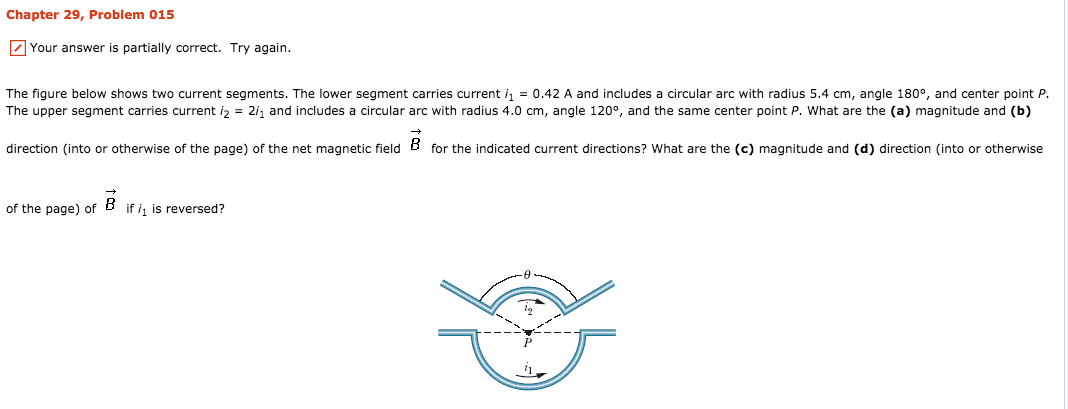# Chapter 29, Problem 015Your answer is partially correct. Try again.The figure below shows two current segments. The lower segment carries current i1 = 0.42 A and includes a circular arc with radius 5.4 cm, angle 180°, and center point P.The upper segment carries current i2 = 2h and includes a circular arc with radius 4.0 cm, angle 120°, and the same center point P, what are the (a) magnitude and (b)direction (into or otherwise of the page) of the net magnetic field B for the indicated current directions? What are the (c) magnitude and (d) direction (into or otherwiseof the page) ofif is reversed?

Question
20 views

I'm trying to study this problem for my exam but when I followed the solution on here I did not get the correct answer in Wiley Plus.help_outlineImage TranscriptioncloseChapter 29, Problem 015 Your answer is partially correct. Try again. The figure below shows two current segments. The lower segment carries current i1 = 0.42 A and includes a circular arc with radius 5.4 cm, angle 180°, and center point P. The upper segment carries current i2 = 2h and includes a circular arc with radius 4.0 cm, angle 120°, and the same center point P, what are the (a) magnitude and (b) direction (into or otherwise of the page) of the net magnetic field B for the indicated current directions? What are the (c) magnitude and (d) direction (into or otherwise of the page) of if is reversed? fullscreen
check_circle

Step 1

(a)

The net magnetic field at the point P is given by,

Step 2

Substituting the values,

The magnitude of the magnetic field is. -1.95×10-6 T

Step 3

(b)

The direction of the net magnetic...

### Want to see the full answer?

See Solution

#### Want to see this answer and more?

Solutions are written by subject experts who are available 24/7. Questions are typically answered within 1 hour.*

See Solution
*Response times may vary by subject and question.
Tagged in